# The Azimuth Project Blog - network theory (part 6)

This page is a blog article in progress, written by John Baez. To see the final polished article, go to the Azimuth Blog.

Now for the fun part. Let’s see how quantum theory ideas can be used to understand random processes. I’ll try to make this post completely self-contained, except at the very end. So, even if you skipped a bunch of the previous ones, this should make sense.

You also don’t need to know quantum theory, though you’ll have more fun if you do. What we’re doing here is very similar, but also strangely different—for reasons I explained last time.

#### Rabbits and quantum mechanics

Suppose we have a population of rabbits in a cage and we’d like to describe its growth in a stochastic way, using probability theory. Let $\psi_n$ be the probability of having $n$ rabbits. We can borrow a trick from quantum theory, and summarize all these probabilities in a formal power series like this:

$\Psi = \sum_{n = 0}^\infty \psi_n z^n$

The variable $z$ doesn’t mean anything in particular, and we don’t care if the power series converges. See, in math ‘formal’ means “it’s only symbols on the page, just follow the rules”. It’s like when someone says a party is ‘formal’, so you need to wear a white tie: you’re not supposed to ask what the tie means.

However, there’s a good reason for this trick. We can define two operators on formal power series, called the annihilation operator:

$a \Psi = \frac{d}{d z} \Psi$

and the creation operator:

$a^\dagger \Psi = z \Psi$

They’re just differentiation and multiplication by $z$, respectively. So, for example, suppose we start out being 100% sure we have $n$ rabbits for some particular number $n$. Then $\psi_n = 1$, while all the other probabilities are 0, so:

$\Psi = z^n$

If we then apply the creation operator, we obtain

$a^\dagger \Psi = z^{n+1}$

Voilà! One more rabbit!The annihilation operator is more subtle. If we start out with $n$ rabbits:

$\Psi = z^n$

and then apply the annihilation operator, we obtain

$a \Psi = n z^{n-1}$

What does this mean? The $z^{n-1}$ means we have one fewer rabbit than before. But what about the factor of $n$? It means there were $n$ different ways we could pick a rabbit and make it disappear! This should seem a bit mysterious, for various reasons… but we’ll see how it works soon enough.

The creation and annihilation operators don’t commute:

$(a a^\dagger - a^\dagger a) \Psi = \frac{d}{d z} (z \Psi) - z \frac{d}{d z} \Psi = \Psi$

so for short we say:

$a a^\dagger - a^\dagger a = 1$

or even shorter:

$[a, a^\dagger] = 1$

where the commutator of two operators is $[S,T] = S T - T S$.

The noncommutativity of operators is often claimed to be a special feature of quantum physics, and the creation and annihilation operators are fundamental to understanding the quantum harmonic oscillator. There, instead of rabbits, we’re studying quanta of energy, which are peculiarly abstract entities obeying rather counterintuitive laws. So, it’s cool that the same math applies to purely classical entities, like rabbits!

Here’s how it works. We want to describe how the probabilities $\psi_n$ change with time, so we write

$\Psi(t) = \sum_{n = 0}^\infty \psi_n(t) z^n$

Then, we write down an equation describing the rate of change of $\Psi$:

$\frac{d}{d t} \Psi(t) = H \Psi(t)$

Here $H$ is an operator called the Hamiltonian, and the equation is called the master equation. The details of the Hamiltonian depend on our problem! But we can often write it down using creation and annihilation operators. Let’s do some examples, and then I’ll tell you the general rule.

#### Catching rabbitsLast time I told you what happens when we stand in a river and catch fish as they randomly swim past. Today we’re doing rabbits. This works almost the same way. But there’s a fundamental difference: rabbits don’t swim.

So, suppose an inexhaustible supply of rabbits are randomly roaming around a huge field, and each time a rabbit enters a certain area, we catch it and add it to our population of caged rabbits. Suppose that on average we catch one rabbit per unit time. Suppose the chance of catching a rabbit during any interval of time is independent of what happens before or afterwards. What is the Hamiltonian describing the probability distribution of caged rabbits, as a function of time?

There’s an obvious dumb guess: the creation operator! However, we saw last time that this doesn’t work, and we saw how to fix it. The right answer is

$H = a^\dagger - 1$

To see why, suppose for example that at some time $t$ we have $n$ rabbits, so:

$\Psi(t) = z^n$

Then the master equation says that at this moment,

$\frac{d}{d t} \Psi(t) = (a^\dagger - 1) \Psi(t) = z^{n+1} - z^n$

Since $\Psi = \sum_{n = 0}^\infty \psi_n(t) z^n$, this implies that the coefficients of our formal power series are changing like this:

$\frac{d}{d t} \psi_{n+1}(t) = 1$
$\frac{d}{d t} \psi_{n}(t) = -1$

while all the rest have zero derivative at this moment. And that’s exactly right! See, $\psi_{n+1}(t)$ is the probability of having one more rabbit, and this is going up at rate 1. Meanwhile, $\psi_n(t)$ is the probability of having $n$ rabbits, and this is going down at the same rate.

Puzzle 1. Show that with this Hamiltonian, the master equation predicts that the expected number of rabbits grows linearly.

#### Dying rabbits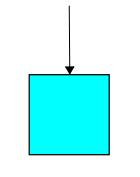Don’t worry: no rabbits are actually injured in the research that Jacob Biamonte is doing here at the Centre for Quantum Technologies. He’s keeping them well cared for in a big room on the 6th floor. This is just a thought experiment.

Suppose a mean nasty guy had a population of rabbits in a cage and didn’t feed them at all. Suppose that each rabbit has a unit probability of dying per unit time. And as always, suppose the probability of this happening in any interval of time is independent of what happens before or after that time.

What is the Hamiltonian? Again there’s a dumb guess: the annihilation operator! And again this guess is wrong, but it’s not far off. As before, the right answer includes a ‘correction term’:

$H = a - N$

This time the correction term is famous in its own right. It’s called the number operator:

$N = a^\dagger a$

The reason is that if we start with $n$ rabbits, and apply this operator, it amounts to multiplication by $n$:

$N z^n = z \frac{d}{d z} z^n = n z^n$

Let’s see why this guess is right. Again, suppose that at some particular time $t$ we have $n$ rabbits, so

$\Psi(t) = z^n$

Then the master equation says that at this time

$\frac{d}{d t} \Psi(t) = (a - N) \Psi(t) = n z^{n-1} - n z^n$

So, our probabilities are changing like this:

$\frac{d}{d t} \psi_{n-1}(t) = n$
$\frac{d}{d t} \psi_n(t) = -n$

while the rest have zero derivative. And this is good! We’re starting with $n$ rabbits, and each has a unit probability per unit time of dying. So, the chance of having one less should be going up at rate $n$. And the chance of having the same number we started with should be going down at the same rate.

Puzzle 2. Show that with this Hamiltonian, the master equation predicts that the expected number of rabbits decays exponentially.

#### Breeding rabbits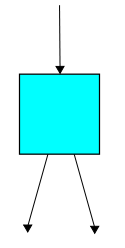Suppose we have a strange breed of rabbits that reproduce asexually. Suppose that each rabbit has a unit probability per unit time of having a baby rabbit, thus effectively duplicating itself.

As you can see from the cryptic picture above, this ‘duplication’ process takes one rabbit as input and has two rabbits as output. So, if you’ve been paying attention, you should be ready with a dumb guess for the Hamiltonian: $a^\dagger a^\dagger a$. This operator annihilates one rabbit and then creates two!

But you should also suspect that this dumb guess will need a ‘correction term’. And you’re right! As always, the correction terms makes the probability of things staying the same go down at exactly the rate that the probability of things changing goes up.

You should guess the correction term… but I’ll just tell you:

$H = a^\dagger a^\dagger a - N$

We can check this in the usual way, by seeing what it does when we have $n$ rabbits:

$H z^n = z^2 \frac{d}{d z} z^n - n z^n = n z^{n+1} - n z^n$

That’s good: since there are $n$ rabbits, the rate of rabbit duplication is $n$. This is the rate at which the probability of having one more rabbit goes up… and also the rate at which the probability of having $n$ rabbits goes down.

Puzzle 3. Show that with this Hamiltonian, the master equation predicts that the expected number of rabbits grows exponentially.

#### Dueling rabbits

Let’s do some stranger examples, just so you can see the general pattern.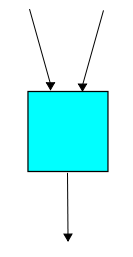Here each pair of rabbits has a unit probability per unit time of fighting a duel with only one survivor. You might guess the Hamiltonian $a^\dagger a a$, but in fact:

$H = a^\dagger a a - N(N-1)$

Let’s see why this is right! Let’s see what it does when we have $n$ rabbits:

$H z^n = z \frac{d^2}{d z^2} z^n - n(n-1)z^n = n(n-1) z^{n-1} - n(n-1)z^n$

That’s good: since there are $n(n-1)$ ordered pairs of rabbits, the rate at which duels take place is $n(n-1)$. This is the rate at which the probability of having one less rabbit goes up… and also the rate at which the probability of having $n$ rabbits goes down.

(If you prefer unordered pairs of rabbits, just divide the Hamiltonian by 2. We should talk about this more, but not now.)

#### Brawling rabbits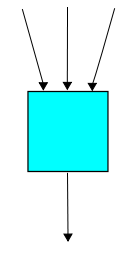Now each triple of rabbits has a unit probability per unit time of getting into a fight with only one survivor! I don’t know the technical term for a three-way fight, but perhaps it counts as a small ‘brawl’ or ‘melee’. In fact the Wikipedia article for ‘melee’ shows three rabbits in suits of armor, fighting it out:Now the Hamiltonian is:

$H = a^\dagger a^3 - N(N-1)(N-2)$

You can check that:

$H z^n = n(n-1)(n-2) z^{n-2} - n(n-1)(n-2) z^n$

and this is good, because $n(n-1)(n-2)$ is the number of ordered triples of rabbits. You can see how this number shows up from the math, too:

$a^3 z^n = \frac{d^3}{d z^3} z^n = n(n-1)(n-2) z^{n-3}$

#### The general rule

Suppose we have a process taking $k$ rabbits as input and having $j$ rabbits as output: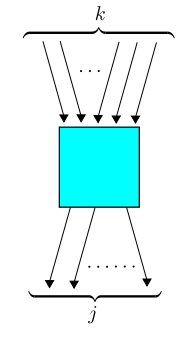I hope you can guess the Hamiltonian I’ll use for this:

$H = {a^{\dagger}}^j a^k - N(N-1) \cdots (N-k+1)$

This works because

$a^k z^n = \frac{d^k}{d z^k} z^n = n(n-1) \cdots (n-k+1) z^{n-k}$

so that if we apply our Hamiltonian to $n$ rabbits, we get

$H z^n = n(n-1) \cdots (n-k+1) (z^{n+j-k} - z^n)$

See? As the probability of having $n+j-k$ rabbits goes up, the probability of having $n$ rabbits goes down, at an equal rate. This sort of balance is necessary for $H$ to be a sensible Hamiltonian in this sort of stochastic theory (an ‘infinitesimal stochastic operator’, to be precise). And the rate is exactly the number of ordered $k$-tuples taken from a collection of $n$ rabbits. This is called the $k$th falling power of $n$, and written as follows:

$n^{\underline{k}} = n(n-1) \cdots (n-k+1)$

Since we can apply functions to operators as well as numbers, we can write our Hamiltonian as:

$H = {a^{\dagger}}^j a^k - N^{\underline{k}}$

#### Kissing rabbits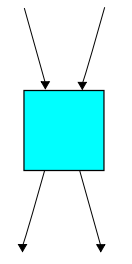Let’s do one more example just to test our understanding. This time each pair of rabbits has a unit probability per unit time of bumping into one another, exchanging a friendly kiss and walking off. This shouldn’t affect the rabbit population at all! But let’s follow the rules and see what they say.

According to our rules, the Hamiltonian should be:

$H = {a^{\dagger}}^2 a^2 - N(N-1)$

However,

${a^{\dagger}}^2 a^2 z^n = z^2 \frac{d^2}{dz^2} z^n = n(n-1) z^n = N(N-1) z^n$

and since $z^n$ form a ‘basis’ for the formal power series, we see that:

${a^{\dagger}}^2 a^2 = N(N-1)$

so in fact:

$H = 0$

That’s good: if the Hamiltonian is zero, the master equation will say

$\frac{d}{d t} \Psi(t) = 0$

so the population, or more precisely the probability of having any given number of rabbits, will be constant.

There’s another nice little lesson here. Copying the calculation we just did, it’s easy to see that:

${a^{\dagger}}^k a^k = N^{\underline{k}}$

This is a cute formula for falling powers of the number operator in terms of annihilation and creation operators. It means that for the general transition we saw before:we can write the Hamiltonian in two equivalent ways:

$H = {a^{\dagger}}^j a^k - N^{\underline{k}} = {a^{\dagger}}^j a^k - {a^{\dagger}}^k a^k$

Okay, that’s it for now! We can, and will, generalize all this stuff to stochastic Petri nets where there are things of many different kinds—not just rabbits. And we’ll see that the master equation we get matches the answer to the puzzle in Part 4. That’s pretty easy. But first, we’ll have a guest post by Jacob Biamonte, who will explain an example from population biology.

category: blog